# Difference between revisions of "Stiffened equation of state"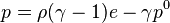$p=\rho(\gamma-1)e-\gamma p^0 \,$
where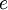$e$ is the internal energy per unit mass,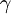$\gamma$ is an empirically determined constant typically taken to be about 6.1, and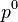$p^0$ is another constant, representing the molecular attraction between water molecules. The magnitude of the later correction is about 2 gigapascals (20,000 atmospheres).
The equation is stated in this form because the speed of sound in water is given by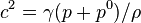$c^2=\gamma(p+p^0)/\rho$.